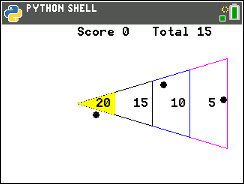# Activities

•• ##### Subject Area

• Math: Explorations with Coding: Coding with Python

6-8

• ##### Device
•TI-84 Plus CE Python

## Shuffleboard Slide#### Activity Overview

In this coding activity for the TI-84 Plus CE Python, students will create a shuffleboard game using Python and mathematics.

#### Objectives

• Use the draw_poly() function to draw triangles
• Use the set_color() function to set pen colors
• Use the randint() function to generate random integers.
• Use if statements to make conditional decisions
• Draw polygons on the coordinate plane
• Use ratios and proportions to transform units
• Decide whether two quantities are proportional
• Use proportions to create scaled polygons